# 基于有限元法的开关磁阻电机抑制振动优化Vibration Suppression Optimization of Switched Reluctance Motor Based on Finite Element Method

DOI: 10.12677/JEE.2019.72013, PDF, HTML, XML, 下载: 421  浏览: 542

Abstract: In order to reduce the vibration and noise of switched reluctance motor (SRM), it has become one of the hot research directions to optimize the structure of the motor. In this paper, a kind of slotting on the rotor teeth is studied, which changes the magnetic field distribution of the rotor teeth, weakens the radial magnetic density and radial force of the air gap, and achieves the purpose of reducing the vibration of the motor. By using the finite element method, a three-phase 12/8 pole SRM with slotted on the rotor teeth is modeled. The slots’ position and width are optimized and the optimal slots sizes are selected. The simulation results show that the structure with slotted on the rotor teeth can optimize the air gap magnetic field, reduce the radial force and suppress the vibration effectively.

1. 引言

2. SRM振动分析

${F}_{n}\left(t\right)=-\frac{1}{2}{i}^{2}\frac{L\left[\theta \left(t\right),{l}_{g}\right]}{{l}_{g}}$ (1)

$T\left(\theta ,i\right)=\frac{1}{2}{i}^{2}\frac{dL}{d\theta }$ (2)

3. SRM的结构Table 1. Main parameters of the motors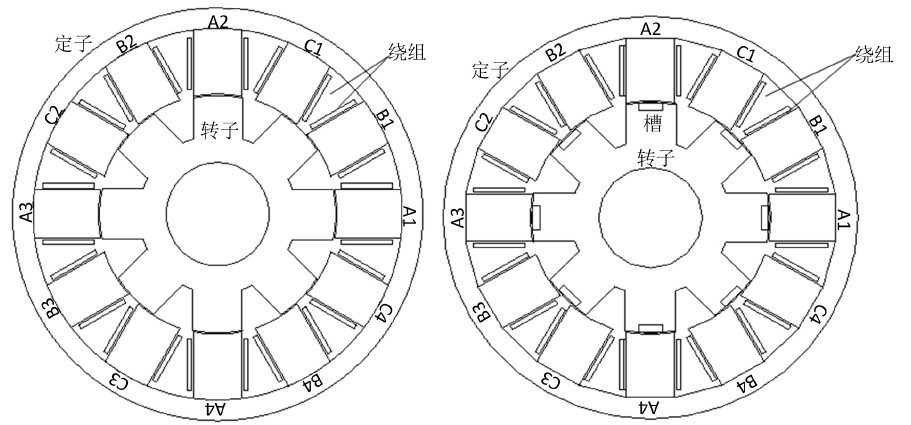(a) (b)

Figure 1. Contrast structure diagram of SRM

4. 有限元仿真分析

4.1. 磁路分析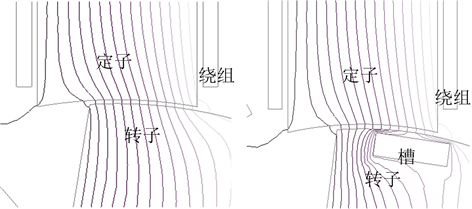(a) (b)

Figure 2. Contrast diagram of air-gap magnetic field; (a) Conventional air-gap magnetic field; (b) Rotor slotted air-gap magnetic field

4.2. 转子槽位置和宽度优化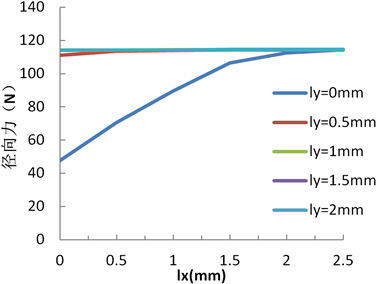Figure 3. Radial force optimization diagram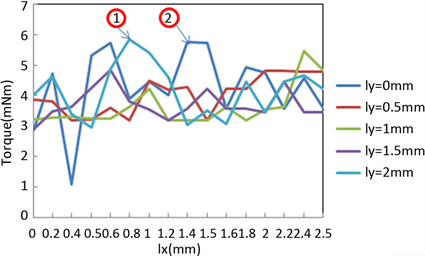Figure 4. Electromagnetic torque optimization diagram

4.3. 瞬态分析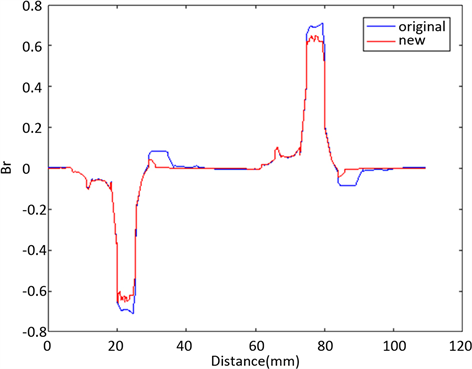Figure 5. Radial magnetic density contrast diagram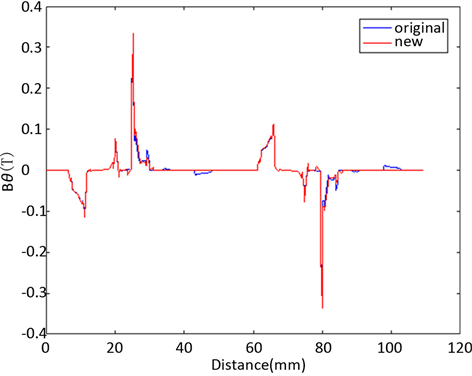Figure 6. Tangential magnetic density contrast diagram

5. 结论

  詹琼华. 开关磁阻电动机[M]. 武汉: 华中理工大学出版社, 1992.  张洁, 裴梓翔. 国内纯电动汽车发展策略分析[J]. 能源与环境, 2017(5): 11-12.  王宏华. 开关磁阻电动机调速控制技术[M]. 北京: 机械工业出版社, 2014: 225-226.  Abramovitz, A. and Ben-Yaakov, S.S. (2018) RGSE Based SPICE Model of Ferrite Core Losses. IEEE Transactions on Power Electronics, 33, 2825-2831. https://doi.org/10.1109/TPEL.2017.2762759  Furqani, J., Kawa, M., Kiyota, K., et al. (2017) Current Waveform for Noise Reduction of Switched Reluctance Motor in Magnetically Saturated Condition. IEEE Transactions on Industry Applications, 54, 213-222. https://doi.org/10.1109/TIA.2017.2756931  Vujicic, V.P. (2017) Minimization of Torque Ripple and Copper Losses in Switched Reluctance Drive. IEEE Transactions on Power Electronics, 27, 388-399. https://doi.org/10.1109/TPEL.2011.2158447  宋桂英, 赵晓明, 邢正坤, 等. 基于多物理场分析开关磁阻电机电磁振动噪声[J]. 微电机, 2017(1): 13-16.  杨光, 杨明发, 石凯. 基于转矩分配的开关磁阻电机转矩脉动抑制的研究[J]. 电工电能新技术, 2017, 36(12): 8-14.  王宏华, 王忠建. 开关磁阻电动机冲击振动特性分析[J]. 中小型电机, 2001, 28(4): 28-31.  吴建华, 孙庆国. 基于转矩分配函数在线修正的开关磁阻电机转矩脉动抑制策略[J]. 电机与控制学报, 2017, 21(12): 8.  张向龙, 杨燕翔, 王军, 等. 开关磁阻电机结构优化现状与发展趋势[J]. 微特电机, 2017, 45(1): 77-80.  陈海东. 开关磁阻电机的设计与振动抑制研究[D]: [硕士学位论文]. 赣州: 江西理工大学, 2018.  王智杰, 蔡燕, 姜文涛. 三相12/8极开关磁阻电机驱动系统建模与仿真[J]. 科技创新与应用, 2017, 3(5): 11.